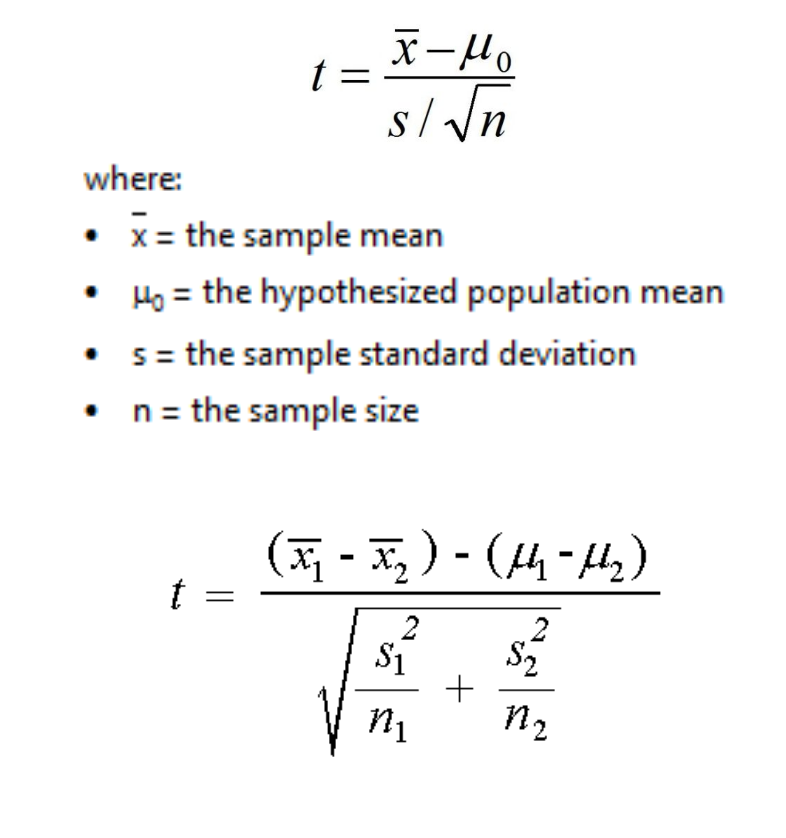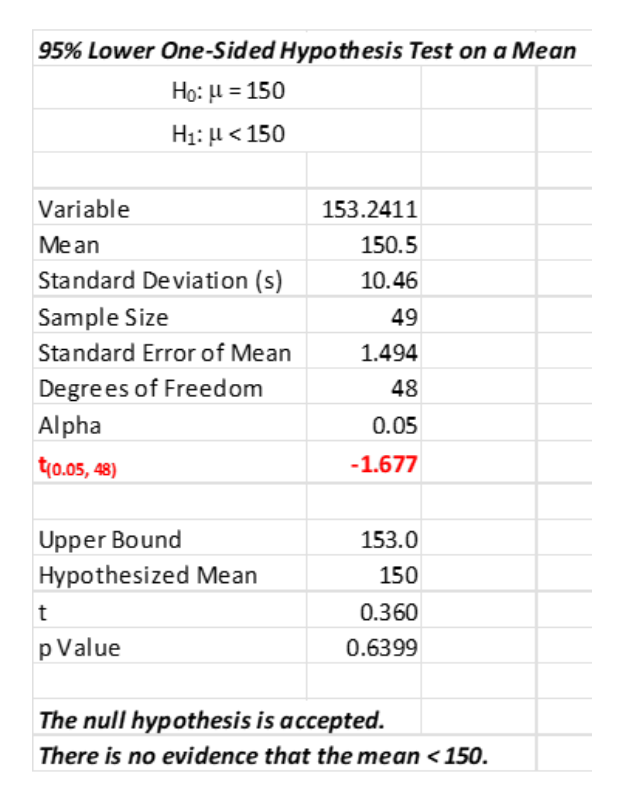The t statistic is a calculation performed during a t-test to determine if you should reject your null hypothesis. You can use a t test to determine if your population is different from some value of interest or whether two samples come from different populations.

## Overview: What is a t statistic?

The t statistic is used in the context of a t-test when doing hypothesis testing. Hypothesis tests start with a null hypothesis (Ho) which states there is no statistically significant difference. An alternative hypothesis (Ha) states there is a statistically significant difference. A one-sample t-test can be used to determine whether your sample comes from a population that is different from some specific value of interest. A 2-sample t-test helps you determine whether two groups of samples are different and whether they come from the same or different populations.

The t-test is used when you have small sample sizes or don’t know the standard deviation of the population. The t statistic for the 1-sample and 2-sample t-tests are some below.## An industry example of a t statistic

The vendor of a newly installed filling machine guaranteed the customer that the new machine would fill/cap/label and pack an average of 150 bottles per minute. The customer’s engineer ran 50 one minute tests over the course of a week. He used a one-sample t-test to see whether the vendor was delivering as promised. The results of the t-test are shown below. Note the t value for the t statistic was relatively small (-1.677)l with an accompanying high p-value (0.6399). The engineer concluded the average fill rate was not less than 150 bottles per hour.### When should I use a t statistic versus a Z statistic?

Use the t statistic when you have small samples or when you don’t know the standard deviation of the population.

### Is a higher or lower value for the t statistic better?

If the value of your t statistic is high, then your p-value will be low. If your p-value is less than your alpha you can reject your null hypothesis and claim something has happened.

### Are there different types of t statistics?

Yes. Depending on the t-test you are using, the formula for calculating the t statistic and the resulting t value will be different.